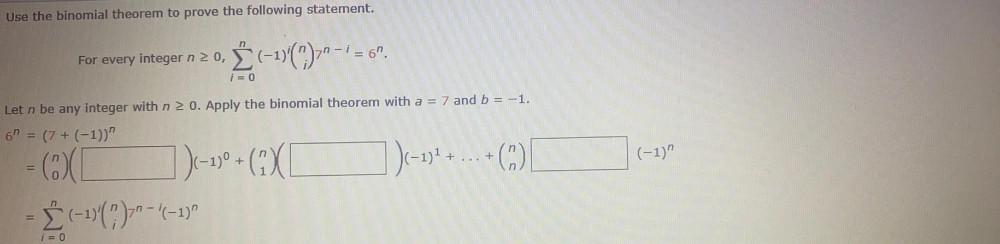Question:

# Use the binomial theorem to prove the following statement. n - For every integer n 20, § (-1)(); - = 6". i = 0 Let n be any inteUse the binomial theorem to prove the following statement. n - For every integer n 20, § (-1)(); - = 6". i = 0 Let n be any integer with n > 0. Apply the binomial theorem with a = 7 and b = -1. 61 = (7 + (-1))" ) ) ( (-1)" + = 0 ) ) ]) (-17 + (2XL) -17% + ... + ( [ - | (-1)();--|(-1) n i = 0